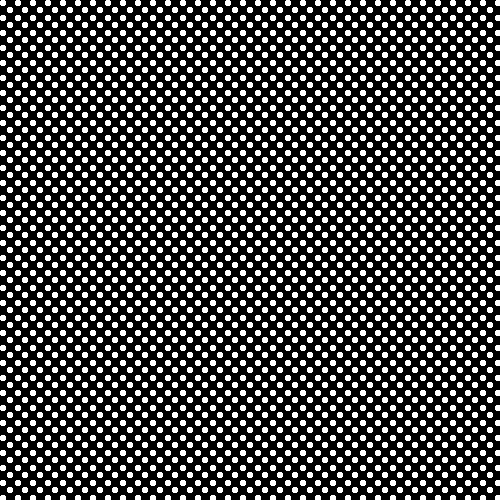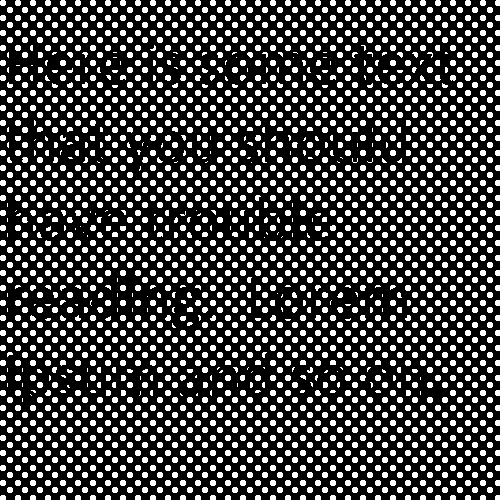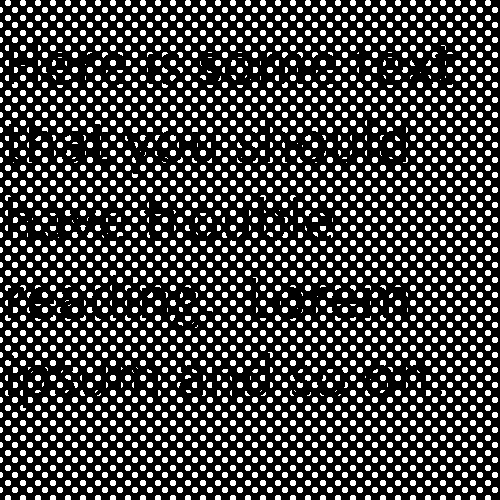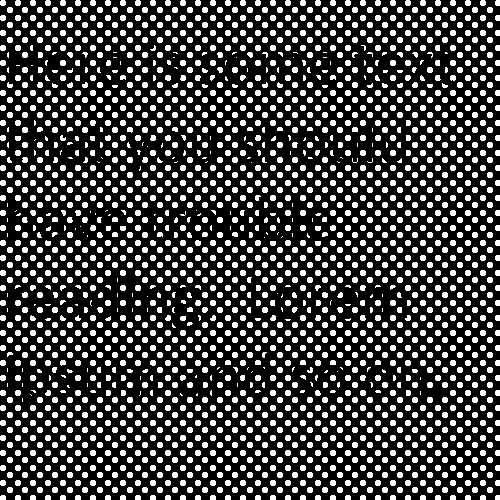Home > Misc

# Looking through a moving perforated screen

In some sort of maybe-not-quite-to-scale form, here is a perforated screen:I was once looking through one of these hole-y screens, and noticed that, when my head was still, I had trouble reading a (relatively) distant sign. But when I moved my head back and forth, reading the sign was easy. Perhaps it was caused by some sort of motion blur, the close screen being blurred and the distant sign unblurred? Or perhaps it needed me to focus on the distant writing, so that the opaque parts of the screen were out of focus. I wondered if I could reproduce the phenomenon on a computer screen, eliminating any actual blur (perhaps there's still some blur in the way the brain processes pictures, I don't know...).

Here is a still of some text behind the screen shown above:And here is the same text, with the screen displaced a couple of different distances:I find the above images difficult-to-impossible to read. But cycle them at 25 frames per second, and the text is almost perfectly clear:I don't really know what the explanation of this phenomenon is. I'm sure it's in some corner of the neuroscience literature, maybe some kind of persistence of vision thing, but I don't know where to start Googl(e-Scholar-)ing. It's interesting to me that it doesn't rely on camera-like blur though, since each still in the above gif is in perfect focus.

R code follows.

``````# References to rows and columns are all backwards because
# EBImage has the opposite convention on referring to
# pixels than what I'm used to.  Luckily for me I made
# square pictures.

library(EBImage)

# text.png should be the same size as the subsequent images created,
# num_rows x num_cols
text_img = text_img[, , 1]
text_img[which(text_img < 0.5)] = 0
text_img[which(text_img > 0)] = 1

# The following as it is just makes a black and white text image,
# but I had a play with making it coloured.
colour_text = array(dim=c(dim(text_img), dim(text_img), 3))
colour_text[, , 1] = text_img
colour_text[, , 2] = text_img
colour_text[, , 3] = text_img

new_text_img = Image(colour_text, colormode=Color)

# r is the radius of the holes in the screen.
r = 3
n = 2*r

# Distance between holes (two sets of holes are created, with
# the second set offset from the first; see code below):
hole_spacing = 15

# Make a circle:
dist_matrix = sqrt((1:n - (r + 0.5))^2 %o% rep(1, n) + rep(1, n) %o% (1:n - (r+0.5))^2)
circle_img = dist_matrix
circle_img[which(dist_matrix > r)] = 1
circle_img[which(dist_matrix <= r)] = 0

# Dimensions of final images:
num_rows = 500
num_cols = 500

# Use a bigger canvas while drawing the circles
# (will truncate later):
nr = num_rows + n + hole_spacing
nc = num_cols + n + hole_spacing

screen_img = matrix(rep(1, nr*nc), nrow=nr, ncol=nc)

# Positions of the first set of holes:
hole_x_seq1 = seq(1, nr, by=hole_spacing)
hole_y_seq1 = seq(1, nc, by=hole_spacing)

offset = floor(hole_spacing/2)

# Positions of the second set of holes:
hole_x_seq2 = hole_x_seq1 + offset
hole_y_seq2 = hole_y_seq1 + offset

# Draw holes:
for (j in 1:length(hole_x_seq1)) {
min_x1 = (j-1)*hole_spacing + 1
max_x1 = min_x1 + n - 1

min_x2 = min_x1 + offset
max_x2 = max_x1 + offset

for (k in 1:length(hole_y_seq1)) {
min_y1 = (k-1)*hole_spacing + 1
max_y1 = min_y1 + n - 1

min_y2 = min_y1 + offset
max_y2 = max_y1 + offset

screen_img[min_y1:max_y1, min_x1:max_x1] = screen_img[min_y1:max_y1, min_x1:max_x1] * circle_img
if ((max_y2 <= nc) & (max_x2 < nr)) {
screen_img[min_y2:max_y2, min_x2:max_x2] = screen_img[min_y2:max_y2, min_x2:max_x2] * circle_img
}
}
}

full_screen_img = screen_img
empty_img = array(dim=c(num_rows, num_cols, 3))

for (ct in 1:hole_spacing) {
screen_img = full_screen_img[ct:(ct+num_rows-1), 1:num_cols]
screen_img = 1 - screen_img
new_img = empty_img
new_img[, , 1] = screen_img
new_img[, , 2] = screen_img
new_img[, , 3] = screen_img

screen_img = Image(new_img, colormode=Color)

main_img = screen_img * new_text_img
outfile = sprintf("images/%03d.png", ct)
writeImage(main_img, outfile)
}```
```

Posted 2014-03-03.

Home > Misc